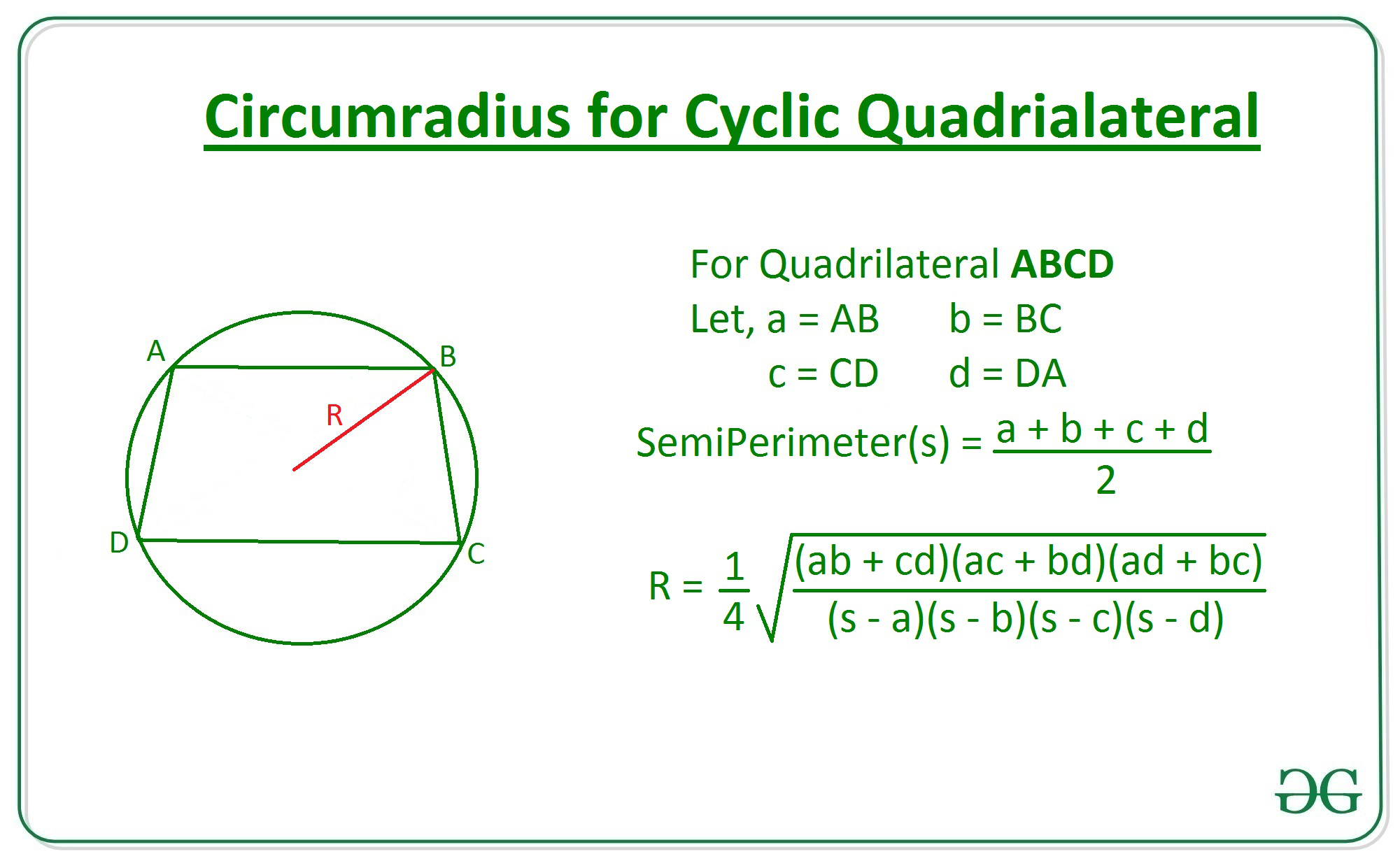Given integers A, B, C, and D denoting the length of sides of a Cyclic Quadrilateral, the task is to find the circumradius i.e., the radius of circumcircle of the given cyclic quadrilateral.

Examples:

Input: A = 3, B = 4, C = 5, D= 6
Output: 3.29

Input: A = 10, B = 30, C = 50, D = 20
Output: 27.78

Approach: Follow the steps below to solve the problem:

• Calculate the semiperimeter of the cyclic quadrilateral with sides A, B, C and D by using the equation:• Now, using Parameshvara’s circumradius formula shown below, calculate the radius for the circumcircle:Below is the implementation of the above approach:

## C++14

 // C++ program to find circumradius of  // a cyclic quadrilateral using sides  #include  using namespace std;     // Function to return the circumradius  // of a cyclic quadrilateral using sides  double Circumradius(int a, int b, int c, int d)  {             // Find semiperimeter      double s = (a + b + c + d) / 2.0;         // Calculate the radius      double radius = sqrt(((a * b) + (c * d)) *                           ((a * c) + (b * d)) *                            ((a * d) + (b * c)) /                            ((s - a) * (s - b) *                             (s - c) * (s - d)));         return radius / 4;  }     // Driver Code  int main()  {      int A = 3;      int B = 4;      int C = 5;      int D = 6;         // Function call      double ans = Circumradius(A, B, C, D);         // Print the radius      cout << setprecision(3) << ans;         return 0;  }     // This code is contributed by mohit kumar 29

## Java

 // Java program to find circumradius of  // a cyclic quadrilateral using sides  import java.util.*;     class GFG{     // Function to return the circumradius  // of a cyclic quadrilateral using sides  static double Circumradius(int a, int b,                              int c, int d)  {             // Find semiperimeter      double s = (a + b + c + d) / 2.0;         // Calculate the radius      double radius = Math.sqrt(((a * b) + (c * d)) *                                ((a * c) + (b * d)) *                                 ((a * d) + (b * c)) /                                 ((s - a) * (s - b) *                                  (s - c) * (s - d)));         return radius / 4;  }     // Driver Code  public static void main(String[] args)  {      int A = 3;      int B = 4;      int C = 5;      int D = 6;         // Function call      double ans = Circumradius(A, B, C, D);         // Print the radius      System.out.format("%.2f", ans);  }  }     // This code is contributed by 29AjayKumar

## Python3

 # Program to find Circumradius of  # a cyclic quadrilateral using sides     import math         # Function to return the Circumradius  # of  a cyclic quadrilateral using sides  def Circumradius(a, b, c, d):         # Find semiperimeter      s = (a + b + c + d) / 2        # Calculate the radius      radius = (1 / 4)*math.sqrt(((a * b)+(c * d))*         ((a * c)+(b * d))*((a * d)+(b * c))          /((s-a)*(s-b)*(s-c)*(s-d)))             return radius         # Driver Code     # Given sides  A = 3 B = 4 C = 5 D = 6      # Function Call    ans = Circumradius(A, B, C, D)         # Print the radius  print(round(ans, 2))

## C#

 // C# program to find circumradius of  // a cyclic quadrilateral using sides  using System;     class GFG{     // Function to return the circumradius  // of a cyclic quadrilateral using sides  static double Circumradius(int a, int b,                              int c, int d)  {             // Find semiperimeter      double s = (a + b + c + d) / 2.0;         // Calculate the radius      double radius = Math.Sqrt(((a * b) + (c * d)) *                                ((a * c) + (b * d)) *                                 ((a * d) + (b * c)) /                                 ((s - a) * (s - b) *                                  (s - c) * (s - d)));         return radius / 4;  }     // Driver Code  public static void Main(String[] args)  {      int A = 3;      int B = 4;      int C = 5;      int D = 6;         // Function call      double ans = Circumradius(A, B, C, D);         // Print the radius      Console.Write("{0:F2}", ans);  }  }     // This code is contributed by 29AjayKumar

Output:

3.29


Time Complexity: O(1)
Auxiliary Space: O(1)

Attention reader! Don’t stop learning now. Get hold of all the important DSA concepts with the DSA Self Paced Course at a student-friendly price and become industry ready.

My Personal Notes arrow_drop_upCheck out this Author's contributed articles.

If you like GeeksforGeeks and would like to contribute, you can also write an article using contribute.geeksforgeeks.org or mail your article to contribute@geeksforgeeks.org. See your article appearing on the GeeksforGeeks main page and help other Geeks.

Please Improve this article if you find anything incorrect by clicking on the "Improve Article" button below.